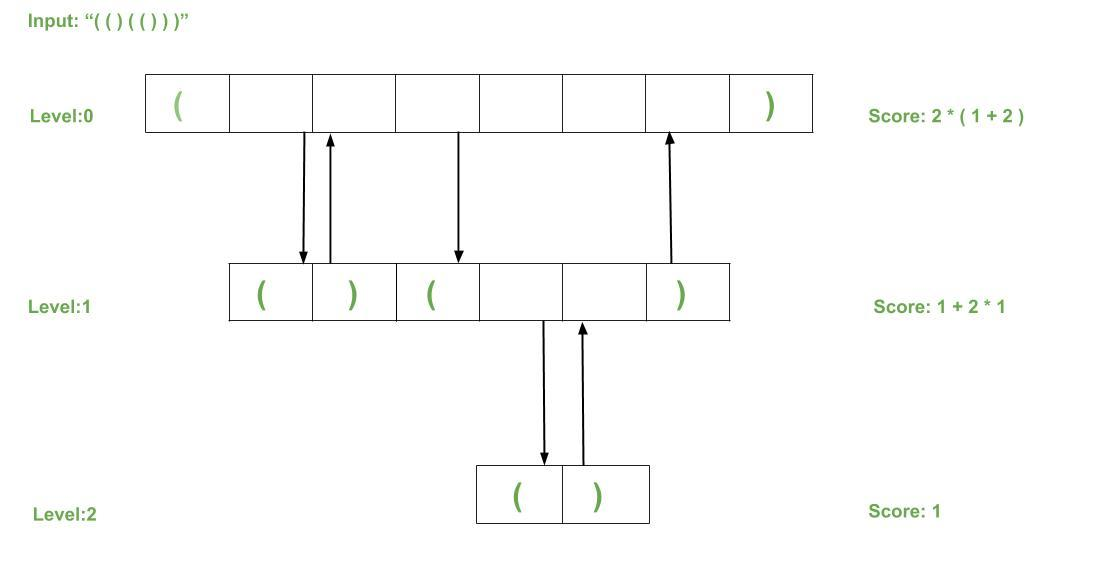# Score of Parentheses using Tree

Given a string str which contains pairs of balanced parentheses, the task is to calculate the score of the given string based on the given rules:

1. “()” has a score of 1.
2. “x y” has a score of x + y where x and y are individual pairs of balanced parentheses.
3. “(x)” has a score twice of x (i.e), the score is 2 * score of x.

Examples:

Input: str = “()()”
Output: 2
Explanation:
Here input is of the form “xy” which makes the total score = score of x + score of y
and hence, score = 1 + 1 = 2

Input: str = “(())”
Output: 2
Explanation:
Here input is of the form “(x)” which makes the total score = 2 * score of x
and hence, score = 2 * 1 = 2

Input: str = “(()()())”
Output: 6
Explanation:
Here input is of the form “(xyz)” which makes the total score = 2 * (score of x +
score of y + score of z) and hence 2*(1 + 1 + 1) = 6

## Recommended: Please try your approach on {IDE} first, before moving on to the solution.

Approach: The idea is to use a tree data structure to solve this problem along with Recursion.

• The root node of our tree structure will represent the outermost pair of our input parentheses.
• For every pair of balanced parentheses included inside the outermost parentheses, we will add a child to our root node.
• This process of declaring a child to a root node will be recursive and hence it will create a node in our tree structure for every pair of balanced parentheses in a hierarchy.
• Every balanced pair of parentheses will be considered as outermost (recursively) and generate a node and hence will allow us to calculate the score.
• When computing score, each leaf node of our tree will be considered with a score of 1 and to get the score of its respective root node we need to add the scores of each child node and double that aggregate.
• The diagram below shows the recursive structure of the tree generated and we start from the bottom to calculate the scores at each level until we reach the outermost ending parentheses.
•Below is the implementation of the above approach:

 `// C++ program to find the score of ` `// parentheses using Tree ` ` `  `#include ` `#include ` ` `  `using` `namespace` `std; ` ` `  `// Customized tree class or struct, ` `// contains all required methods. ` `class` `TreeNode { ` `    ``TreeNode* parent = NULL; ` `    ``vector children; ` ` `  `public``: ` `    ``// Function to add a child into ` `    ``// the list of children ` `    ``void` `addChild(TreeNode* node) ` `    ``{ ` `        ``children.push_back(node); ` `    ``} ` ` `  `    ``// Function to change the parent ` `    ``// pointer to the node passed ` `    ``void` `setParent(TreeNode* node) ` `    ``{ ` `        ``parent = node; ` `    ``} ` ` `  `    ``// Function to return the parent ` `    ``// of the current node ` `    ``TreeNode* getParent() ` `    ``{ ` `        ``return` `parent; ` `    ``} ` ` `  `    ``// Function to compute the score recursively. ` `    ``int` `computeScore() ` `    ``{ ` ` `  `        ``// Base case ` `        ``if` `(children.size() == 0) ` `            ``return` `1; ` ` `  `        ``int` `res = 0; ` ` `  `        ``// Adds scores of all children ` `        ``for` `(TreeNode* curr : children) ` `            ``res += curr->computeScore(); ` ` `  `        ``if` `(parent == NULL) ` `            ``return` `res; ` `        ``else` `            ``return` `2 * res; ` `    ``} ` `}; ` ` `  `// Function to create the tree structure ` `TreeNode* computeTree(string s) ` `{ ` ` `  `    ``TreeNode* current = ``new` `TreeNode(); ` `    ``TreeNode* root = current; ` ` `  `    ``// Creating a node for every "()" ` `    ``for` `(``int` `i = 0; i < s.size(); i++) { ` ` `  `        ``// If we find "(" we add a node as ` `        ``// a child ` `        ``if` `(s[i] == ``'('``) { ` `            ``TreeNode* child = ``new` `TreeNode(); ` `            ``child->setParent(current); ` `            ``current->addChild(child); ` `            ``current = child; ` `        ``} ` ` `  `        ``// On finding ")" which confirms that ` `        ``// a pair is closed, we go back ` `        ``// to the parent ` `        ``else` `{ ` ` `  `            ``current = current->getParent(); ` `        ``} ` `    ``} ` `    ``return` `root; ` `} ` ` `  `// Driver code ` `int` `main() ` `{ ` `    ``string s = ``"(()(()))"``; ` ` `  `    ``// Generating the tree ` `    ``TreeNode* root = computeTree(s); ` ` `  `    ``// Computing the score ` `    ``cout << root->computeScore(); ` `    ``return` `0; ` `} `

Output:

```6
```

Time Complexity: O(N), where N is the length of the input string.
Space Complexity: O(N), where N is the length of the input string.My Personal Notes arrow_drop_upCheck out this Author's contributed articles.

If you like GeeksforGeeks and would like to contribute, you can also write an article using contribute.geeksforgeeks.org or mail your article to contribute@geeksforgeeks.org. See your article appearing on the GeeksforGeeks main page and help other Geeks.

Please Improve this article if you find anything incorrect by clicking on the "Improve Article" button below.

Practice Tags :

Be the First to upvote.

Please write to us at contribute@geeksforgeeks.org to report any issue with the above content.